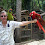## Thursday, April 2, 2015

### Test Yourself: Are You Being Tricked by Intuition?

Not that tough. Think!

1.I got the third one 100% right. I messed up the second one and made a bad assumption in the first.

2.The first one still doesn't make any sense to me.

3.x = cost of ball
cost of bat = x + 1
total cost = 1.10 = x + (x + 1) = 2x + 1
2x = 0.10
x = 0.05

So the ball cost 0.05, the bat costs 1 dollar more than the ball, 1.05, and the total is 1.05 + .05 = 1.10

1.1.10 = 2x + 1
(subtract 1 from both sides of the equation)
0.10 = 2x
(divide both sides by 2)
0.05 = x

4.# for 3!!
Thanks for the coaching, boss, THIMK!!!

5.That was 3 for 3 ooops

6.Yes. Easy if one doesn't leap to any conclusions. Understand the question before the answer.

7.Woo apparently not, 3 out of 3!